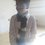# Doubt!

Let $x=1+3a+6a^2+10a^3+\dots$ and $y=1+4b+10b^2+20b^3+\dots$ for $\lvert a\rvert<1$ and $\lvert b\rvert<1$. Find $S=1+3(ab)+5(ab)^2+\dots$ in terms of $x$ and $y$.

I found $x$ and $y$ in terms of $a$ and $b$ respectively, and then substituted in a closed expression for $S$, which came out to be pretty complicated. This leads me to think that I'm wrong somewhere. Also, is there a method faster than finding closed expressions for $x$ and $y$ and then substituting or whatever?Note by Omkar Kulkarni
5 years, 10 months ago

This discussion board is a place to discuss our Daily Challenges and the math and science related to those challenges. Explanations are more than just a solution — they should explain the steps and thinking strategies that you used to obtain the solution. Comments should further the discussion of math and science.

When posting on Brilliant:

• Use the emojis to react to an explanation, whether you're congratulating a job well done , or just really confused .
• Ask specific questions about the challenge or the steps in somebody's explanation. Well-posed questions can add a lot to the discussion, but posting "I don't understand!" doesn't help anyone.
• Try to contribute something new to the discussion, whether it is an extension, generalization or other idea related to the challenge.

MarkdownAppears as
*italics* or _italics_ italics
**bold** or __bold__ bold
- bulleted- list
• bulleted
• list
1. numbered2. list
1. numbered
2. list
Note: you must add a full line of space before and after lists for them to show up correctly
paragraph 1paragraph 2

paragraph 1

paragraph 2

[example link](https://brilliant.org)example link
> This is a quote
This is a quote
    # I indented these lines
# 4 spaces, and now they show
# up as a code block.

print "hello world"
# I indented these lines
# 4 spaces, and now they show
# up as a code block.

print "hello world"
MathAppears as
Remember to wrap math in $$ ... $$ or $ ... $ to ensure proper formatting.
2 \times 3 $2 \times 3$
2^{34} $2^{34}$
a_{i-1} $a_{i-1}$
\frac{2}{3} $\frac{2}{3}$
\sqrt{2} $\sqrt{2}$
\sum_{i=1}^3 $\sum_{i=1}^3$
\sin \theta $\sin \theta$
\boxed{123} $\boxed{123}$

Sort by:

Hint: Differentiate the series $\displaystyle \sum_{n=1}^\infty a^n = \frac a{1-a}$ with respect to $a$.

- 5 years, 10 months ago

Nope, not getting it. Also, we haven't learned differentiation yet, so I can't say I'm familiar enough with derivatives. Could you explain?

- 5 years, 10 months ago

Okay, I will do for $x$. I take it that $1,3,6,10,\ldots$ are all triangular numbers. then $x = \displaystyle \sum_{n=1}^\infty \frac {n(n+1)}2 a^{n-1}$.

If we differentiate $\displaystyle \sum_{n=1}^\infty a^n = \frac a{1-a}$, we get $\displaystyle \sum_{n=1}^\infty na^{n-1} = \frac 1{(1-a)^2 }$. Differentiate once more, we get $\displaystyle \sum_{n=1}^\infty n(n-1)a^{n-2} = \frac 2{(1-a)^3 }$. Then,

\begin{aligned} \displaystyle 2x &=& \sum_{n=1}^\infty n(n+1) a^{n-1} \\ &=& \sum_{n=1}^\infty (n(n-1) + 2n) a^{n-1} \\ &=& \sum_{n=1}^\infty n(n-1) a^{n-1} + 2 \sum_{n=1}^\infty n a^{n-1} \\ &=& a \sum_{n=1}^\infty n(n-1) a^{n-2} + 2 \sum_{n=1}^\infty n a^{n-1} \\ &=& a \cdot \frac2{(1-a)^3} + 2 \cdot \frac1{(1-a)^2} \\ &=& \frac{2a}{(1-a)^3} \\ \end{aligned}

So $x = \frac{a}{(1-a)^3}$. Using the same concept, we can state $y$ in terms of $b$.

Now, a few tricks for $y$: I assume $1,4,10,20,\ldots$ are tetratehedral numbers, that is $T_n = \frac{n(n+1)(n+2)} 6$. Your mission here is to state $n(n+1)(n+2)$ in terms of a linear combination of $n(n-1)(n-2) , n(n-1)$ and $n$ like what I've done above for $x$.

Another thing to note that $S$ is an arithmetic geometric progression.

Can you finish it off?

- 5 years, 10 months ago

Oh okay. But isn't that the same as what I did? Find $a$ in terms of $x$, $b$ in terms of $y$ and substitute in $S$?

- 5 years, 10 months ago

I don't know what method you did, I just input my thoughts on how to solve it.

- 5 years, 10 months ago

Right. Thanks! :D

- 5 years, 10 months ago